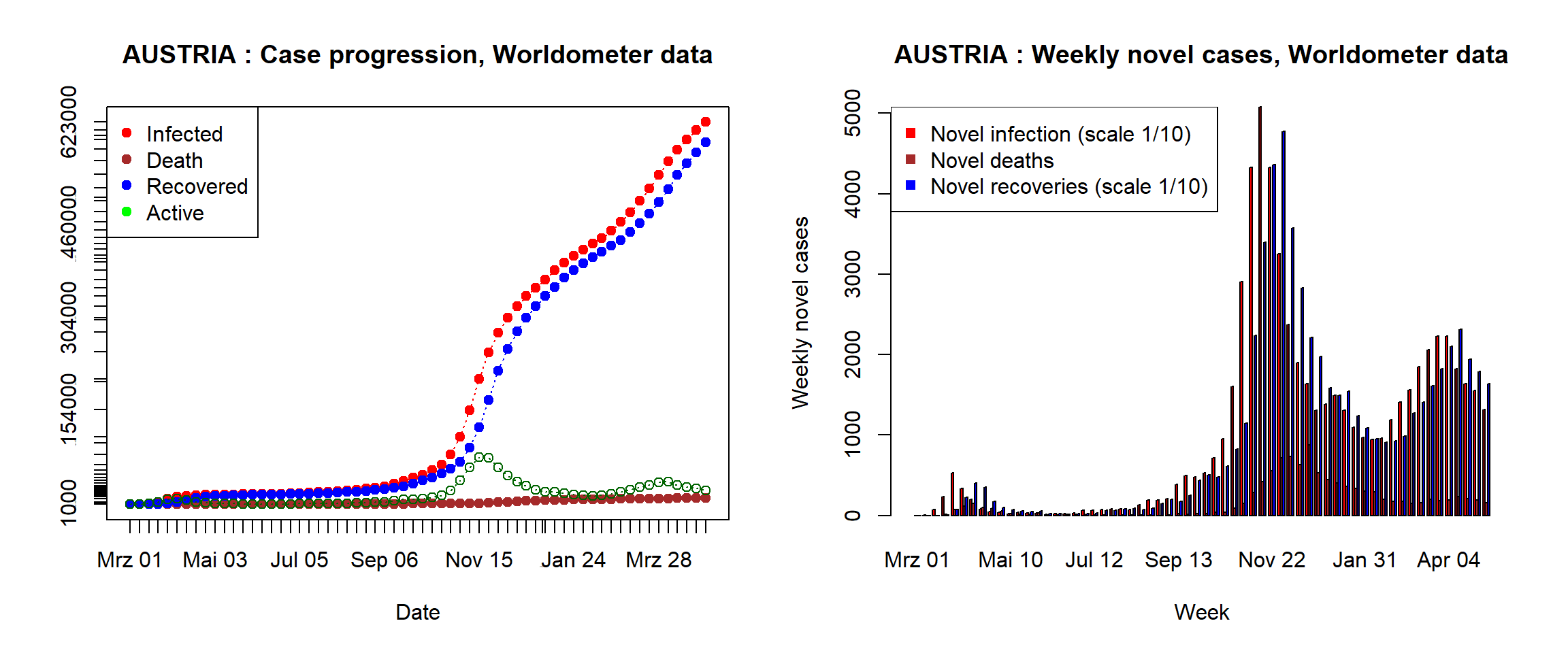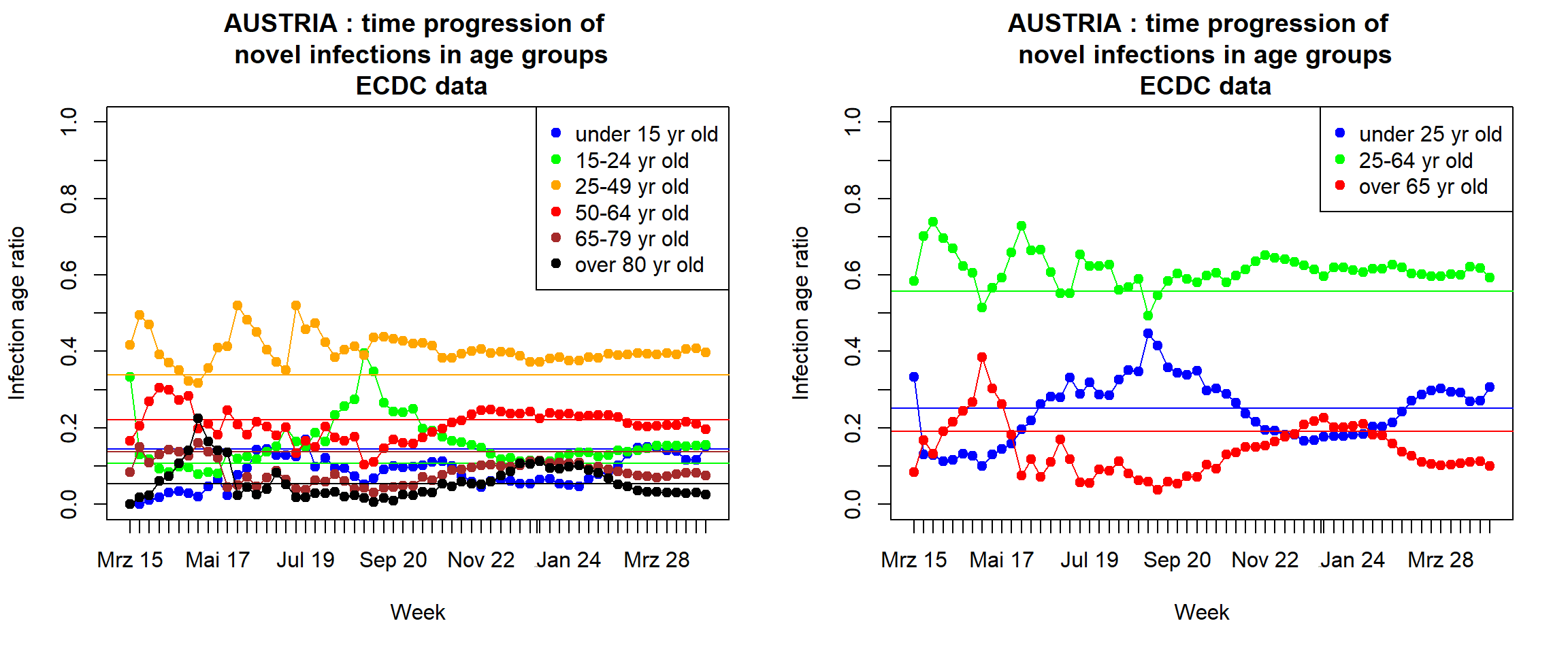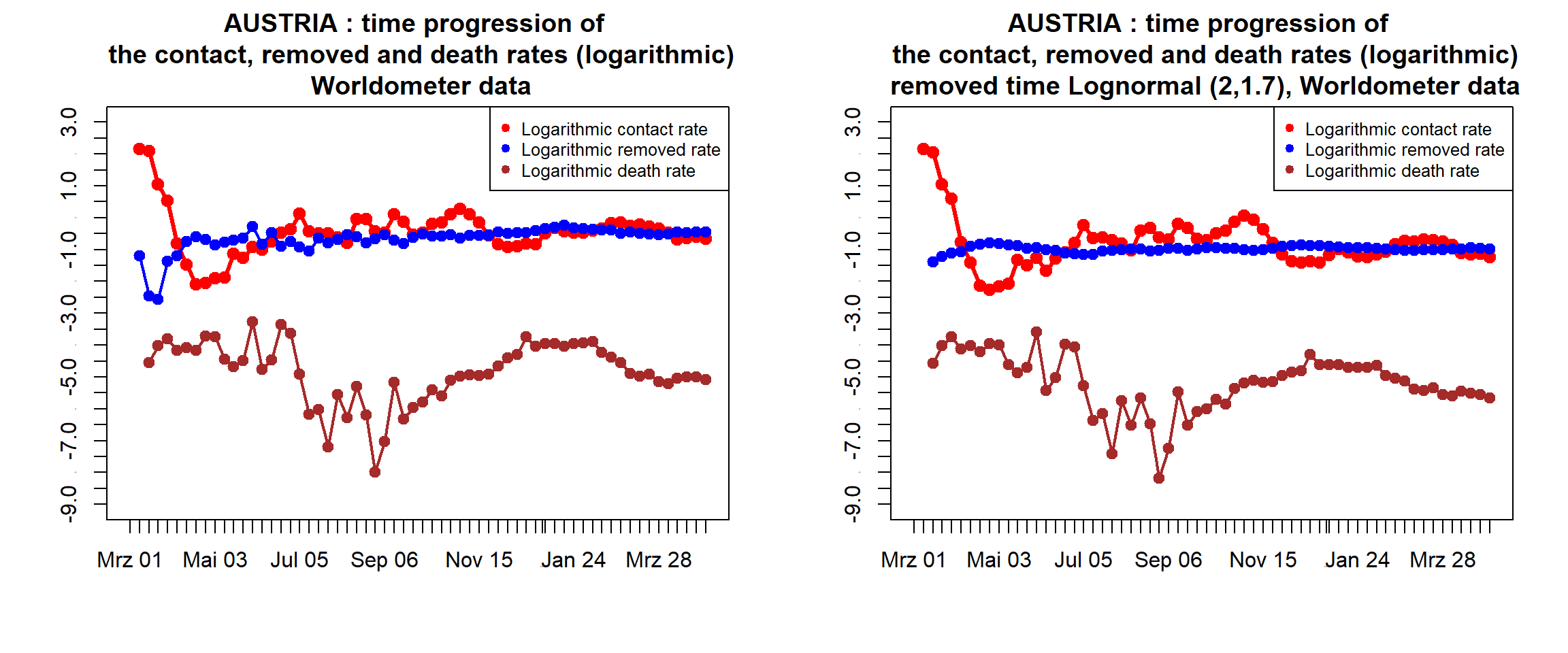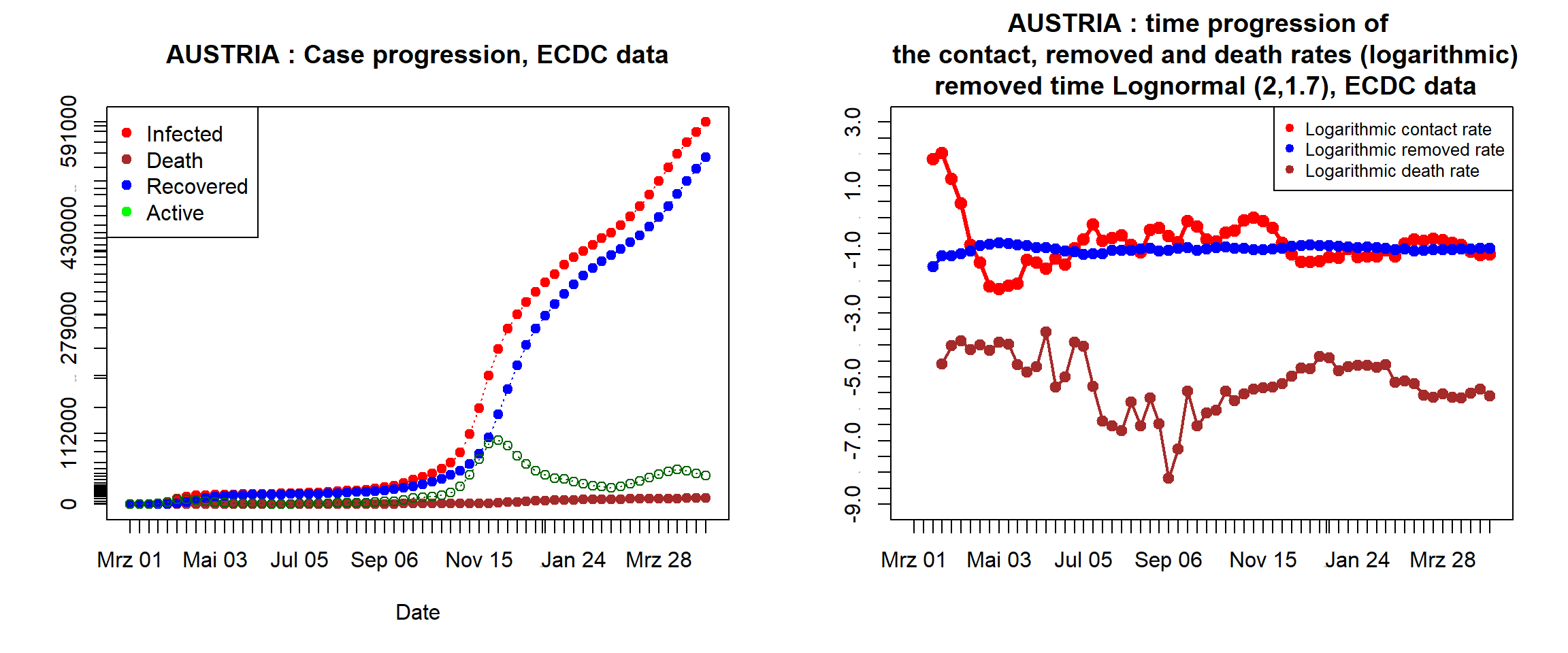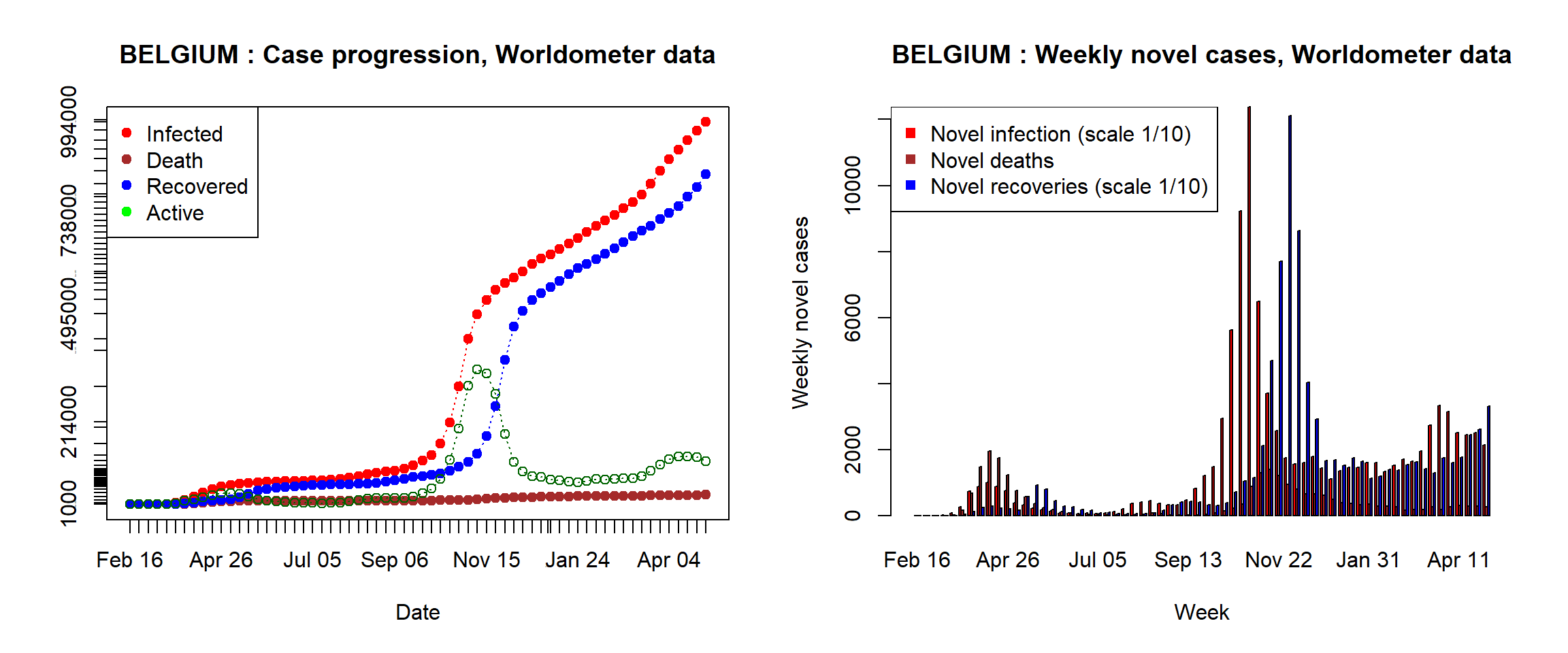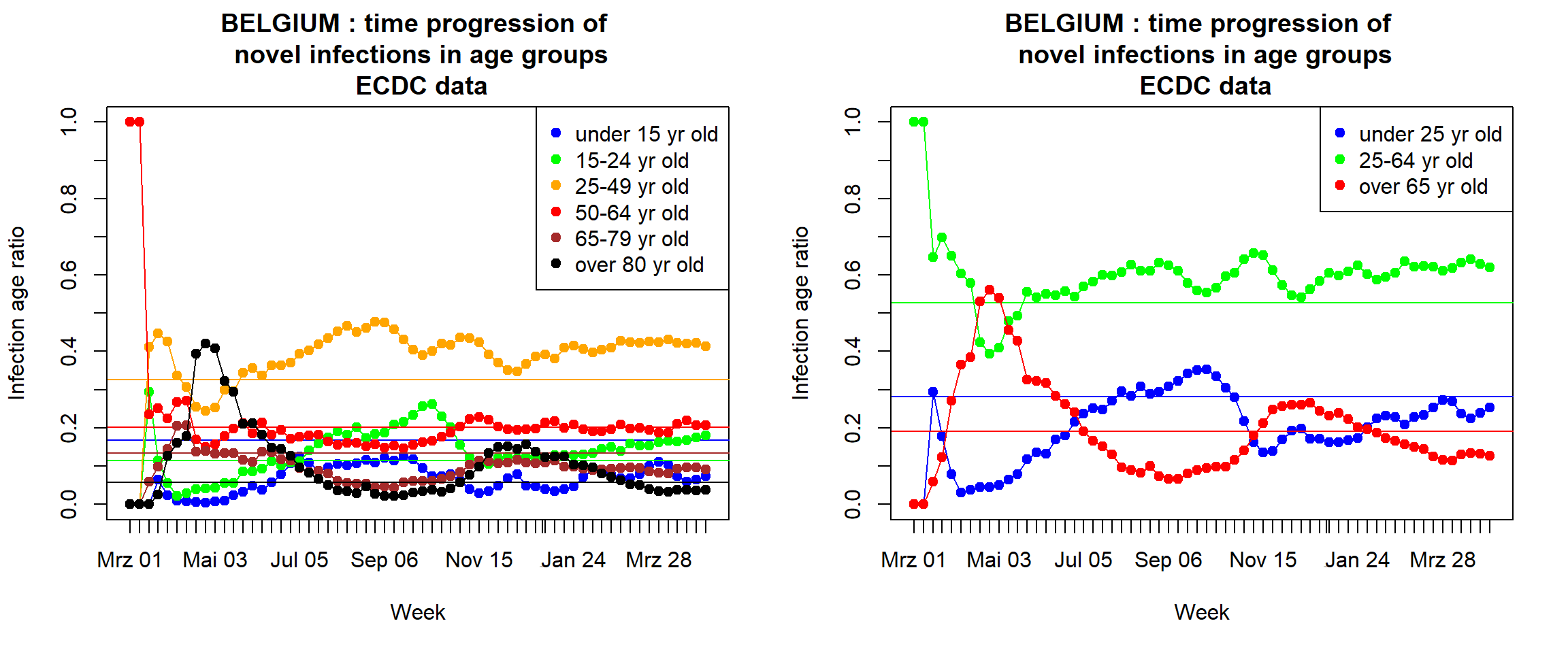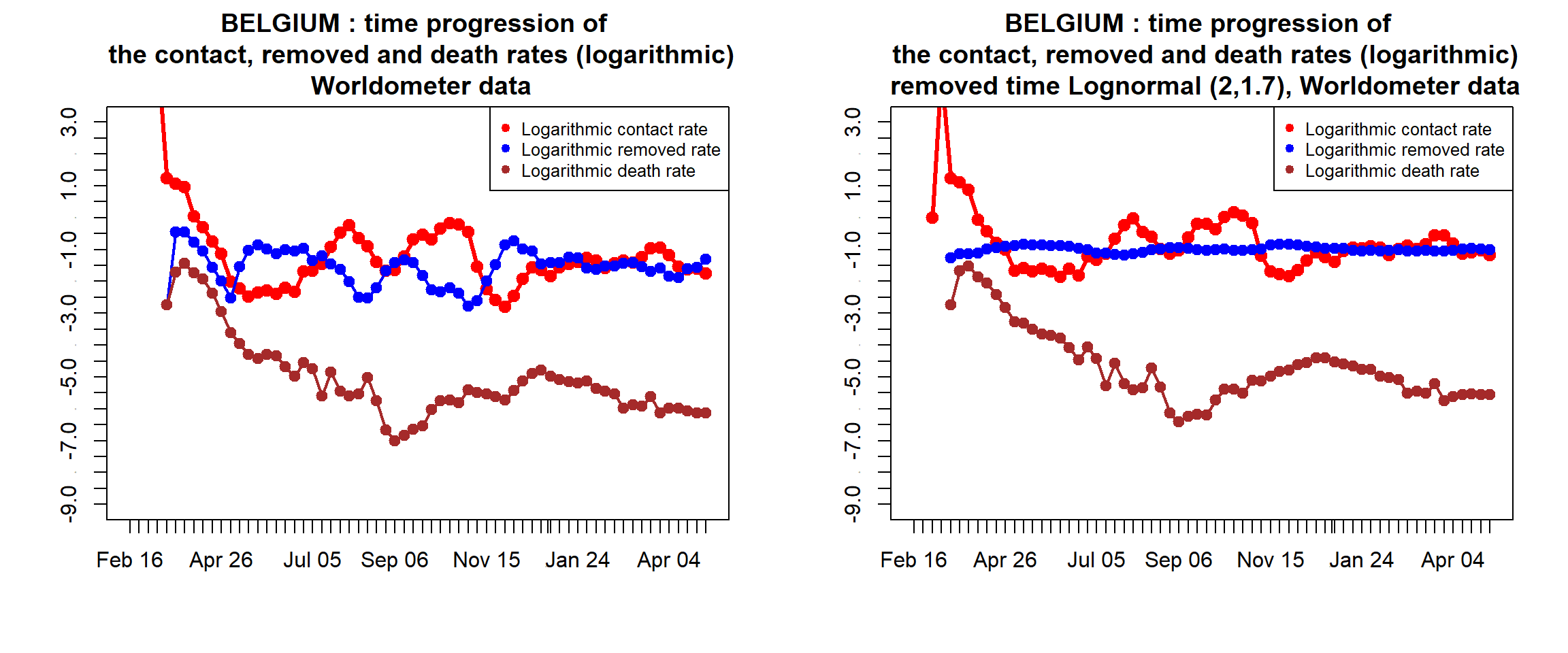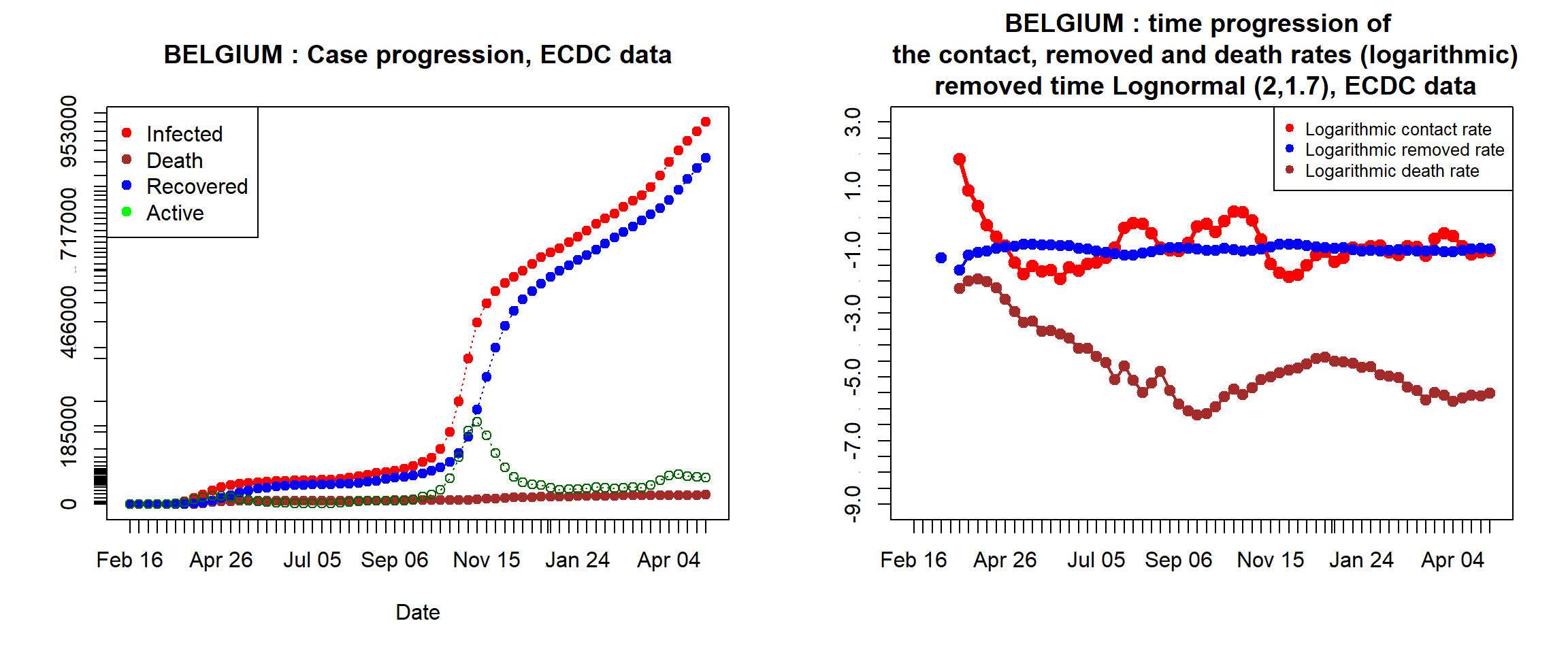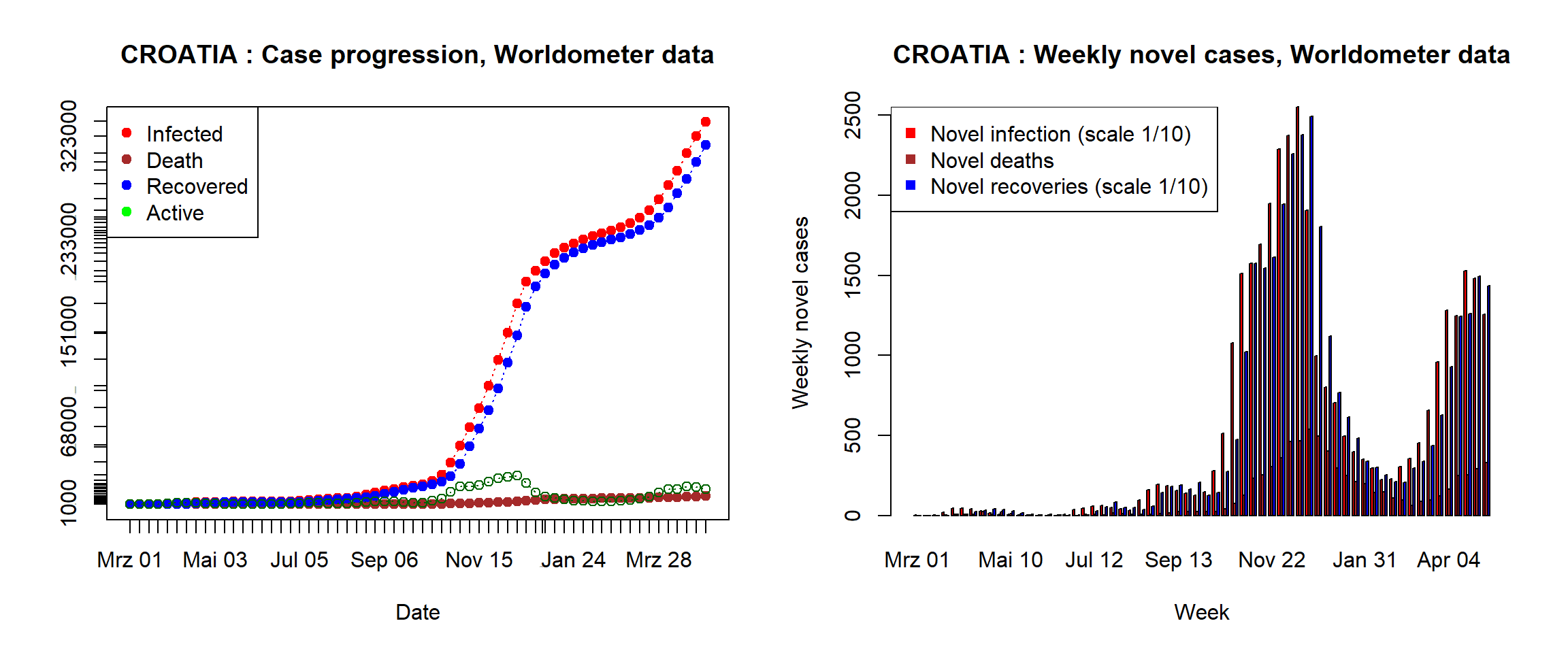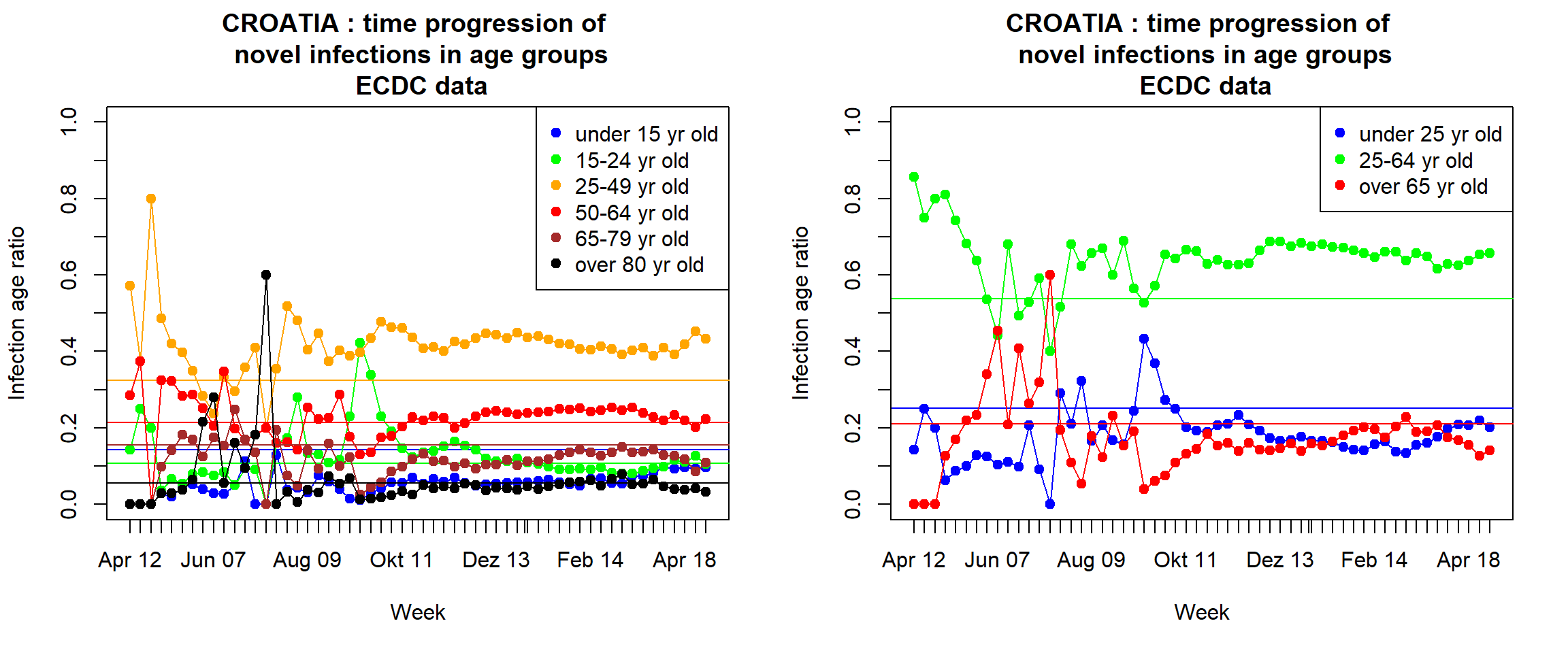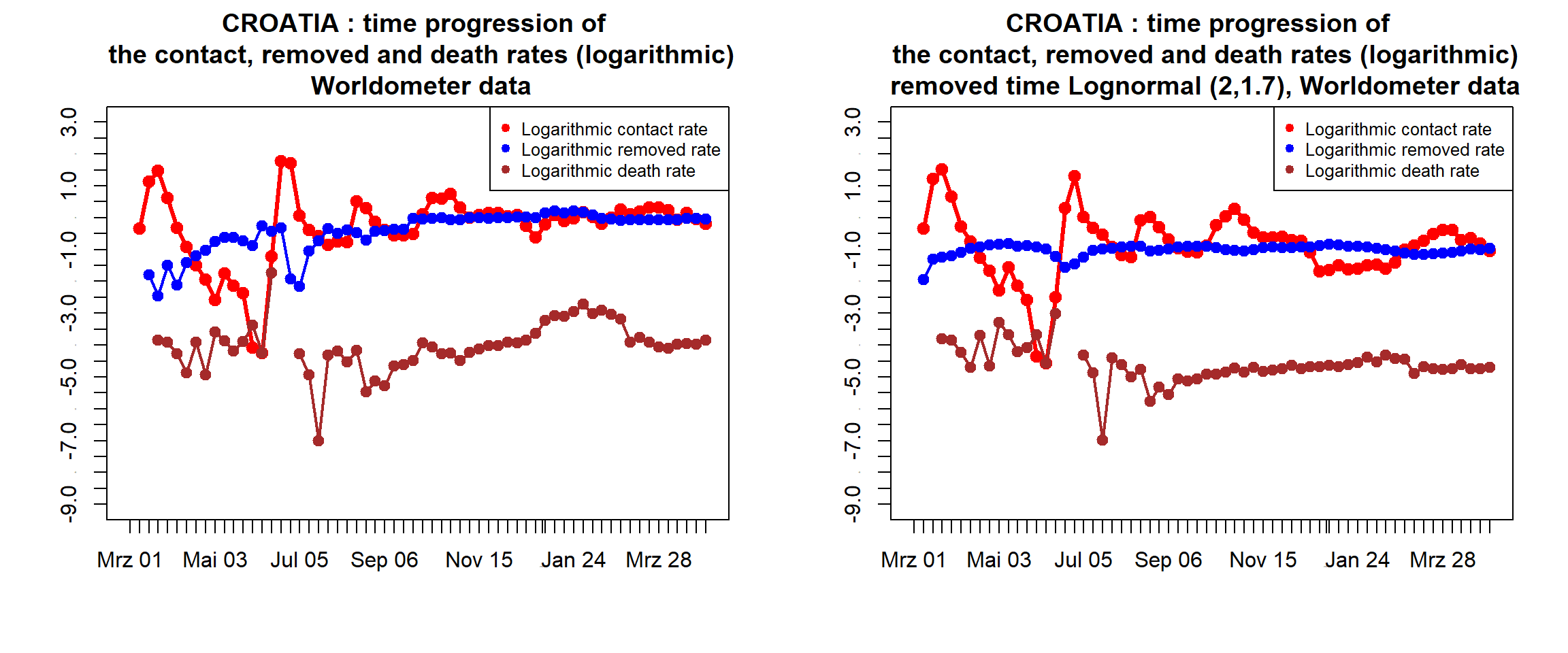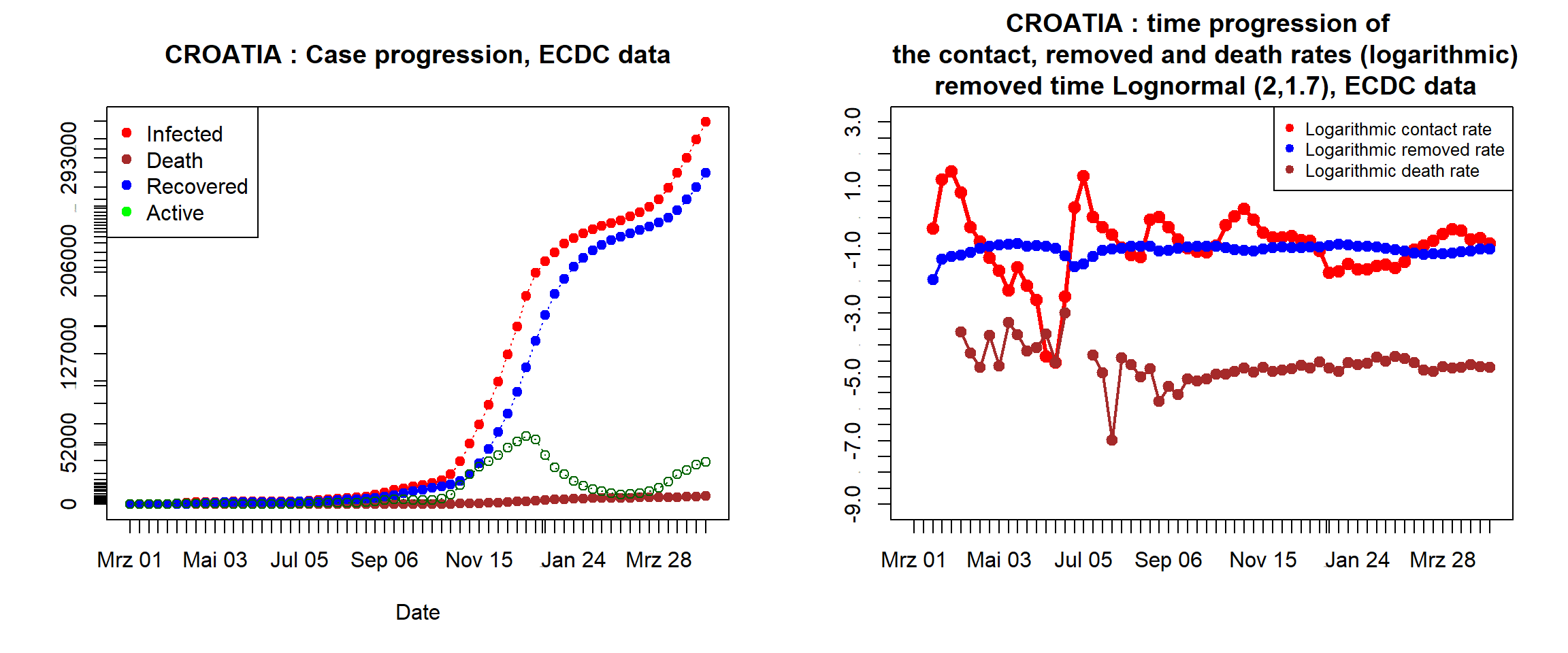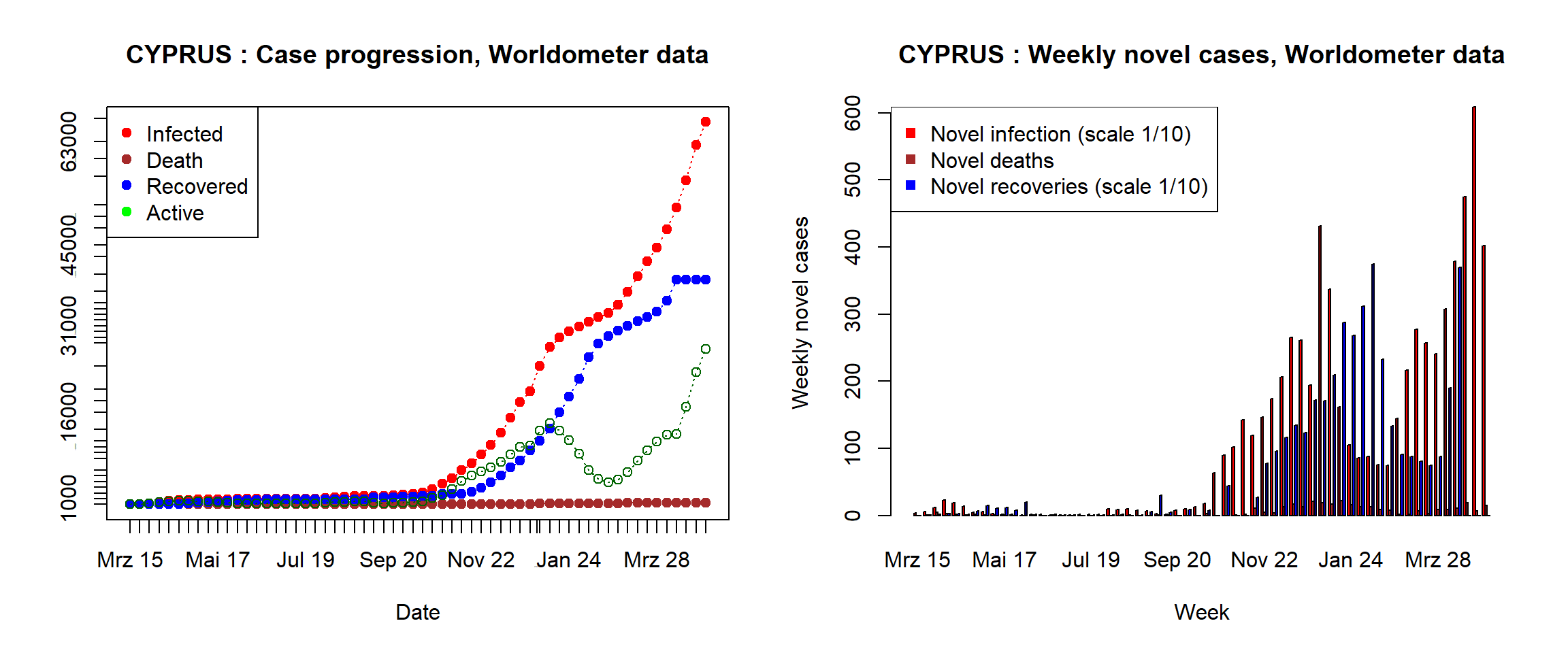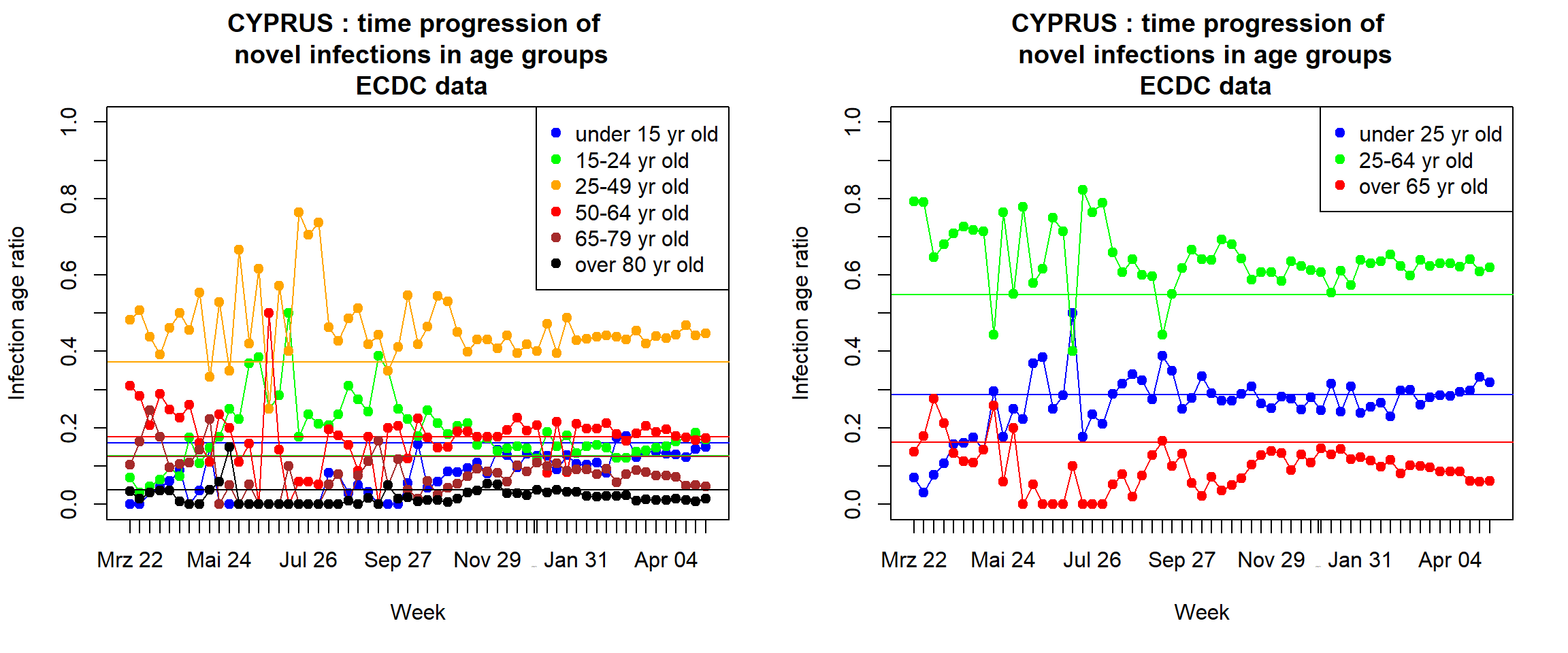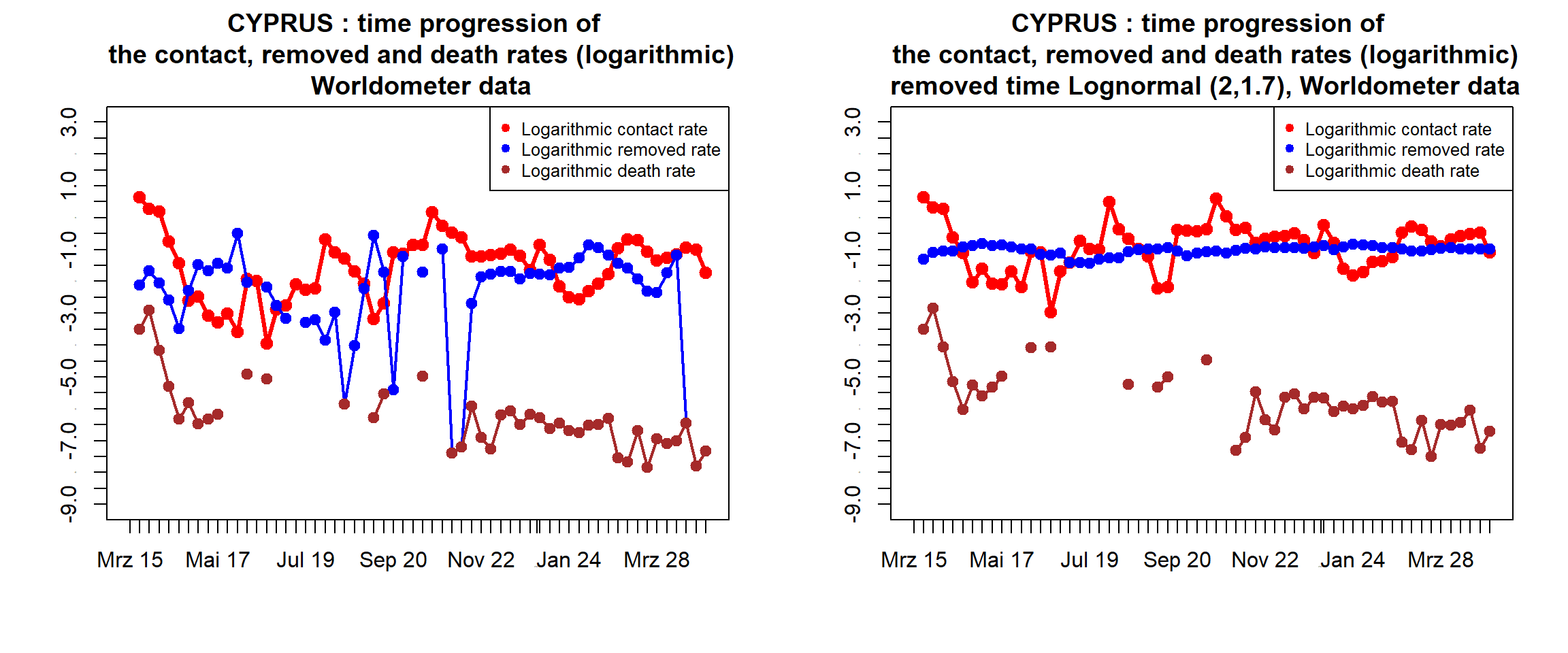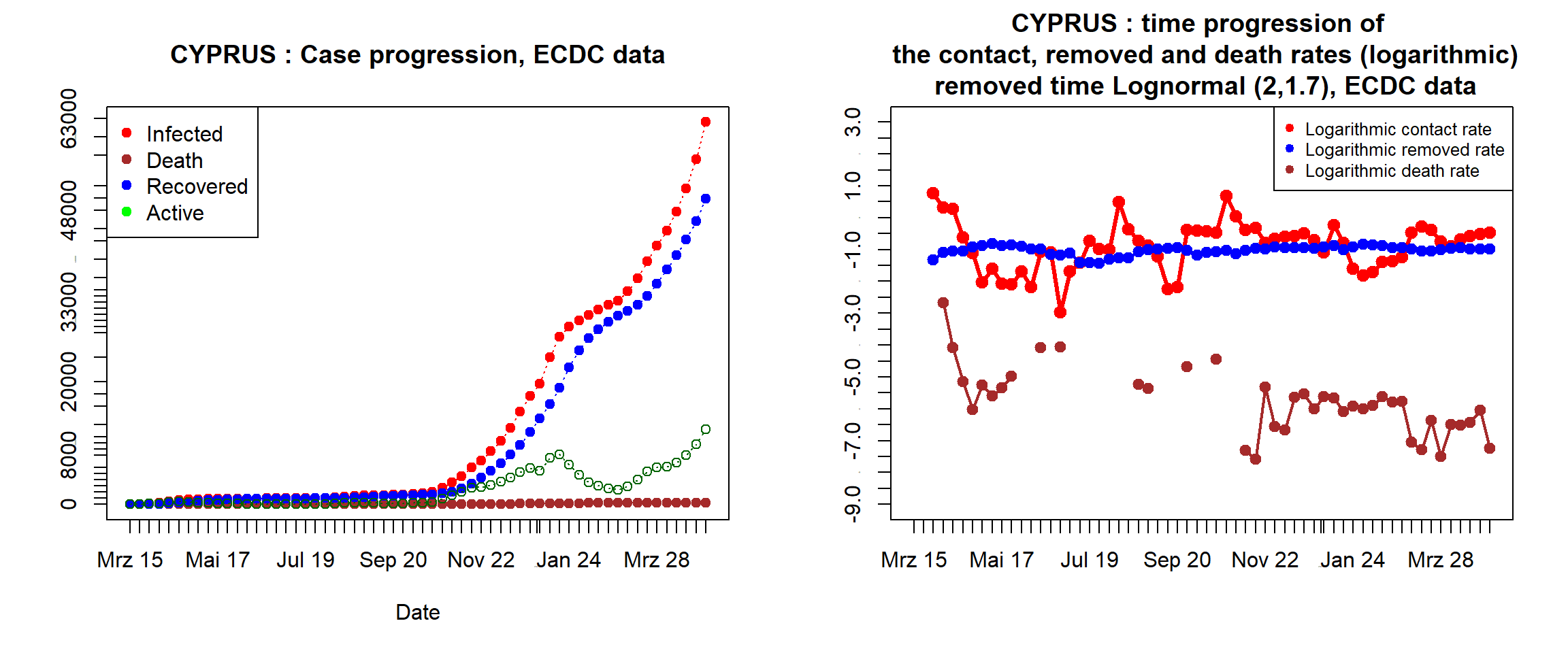# COVID-19 Data Analysis Update - 02. May 2021 - Special report for Europe

### Disclaimer

We evaluate the data provided by

on a weekly basis, concerning the numbers of persons infected, deceased or recovered from the COVID19 virus in different countries. We exclude any liability with regard to the quality and accuracy of the data used, and also with regard to the correctness of the statistical analysis. The evaluations of the different growth phases represent our purely personal opinion. The data are usually based on information provided by national governments or authorities. The number of cases reported may be significantly lower than the number of people actually infected, and these differences may be different in different countries. However, as long as the ratio of reported cases to actual cases remains reasonably constant, statistical trends for actual cases can be derived with all due caution from analysis of the evolution of reported case numbers. In this context, however, we must point out that changes in the test system may lead to fluctuations in reported cases that have no equivalent in actual case numbers. It is also possible that some governments may manipulate their data for political reasons and therefore the reported case numbers may not necessarily correspond to the positively tested cases. Certainly, at this stage all statistical predictions are subject to great uncertainty because the general trends of the epidemic are not yet clear and we do not know to what extent developments in countries where the epidemic appears to be already largely controlled can be transferred other countries. In any case, the statistical trends that we read from the data are only suitable for predictions if the measures taken by the respective governments and authorities to contain the pandemic remain in force and are being followed by the respective populations. We must also point out that, even if the statistics indicate that the epidemic is under control, we may at any time see a resurgence of infection figures until the disease is eradicated worldwide.

### Empirical evidence from Europe

The story can be summarized as follows. COVID-19 started around the end of January 2020 in Europe with several cases detected from people returning from China, but it only became an outbreak at the end of February after the European people returned from the winter holidays in Lombardy and Veneto in Italy. Soon after it became a worldwide pandemic with fast transmission rate and many fatalites, which forced almost all European governments to announce social distancing measures and even national lock-downs in order to contain the spreading of the disease. These policies turned out to be effective and brought the epidemic in Europe under control from the end of April to July 2020, even after the measures were partially relaxed. But as the situation became stable and most of the social distancing policies were lifted at the end of July, people started to travel and transferred the disease. As a result, a second, and much more persistent wave originated roughly around mid July 2020. By the end of October, the numbers of novel daily cases and deaths were substantially higher than during the first wave, forcing all European governments to re-announce social distancing policies and then national lockdowns through Christmas 2020 and the New Year holiday 2021. Thanks to these strict policies, the number of new infections decreased from January 2021 to the lowest level in February 2021. However, as the control measures were relaxed again and new variants of coronavirus from Brazil, England and Africa threatened, a third wave of pandemic happened in the beginning of February 2021 and developed quickly, forcing again the government to go back to partial control measures in March 2021. Hence by the midle of April 2021, the situation in countries like Austria, Belgium, Czech Republic, Estonia, Hungary, Italy, Malta, Poland, Portugal, Romania, and Slovakia is basically in control, while for the other countries in Europe things should be watched closely with care. Picture 1 shows the case progression, while picture 2 plots the weekly novel cases in European countries.

Picture 3 plots the age distribution of the novel infections as time series, and compare them with the age distribution of the total population. In many countries (Belgium, Croatia, Cyprus, Czech Republic, Greece, Italy, Latvia, Luxembourg, Malta, Poland, and Romania), one sees a phenomenon that starting from wave 2, most curves approach horizontal lines of the same age in the total population. When we assume that the spread of the coronavirus is fully mixed in the whole population, the Birkhorff ergodic theorem provides a possible explanation.

In particular in some countries (like Austria, Denmark, Finland, Germany, Netherlands, Norway, Sweden) however, one can see that the ratio of the newly infected group of over 65 year old people fluctuates significantly below the corresponding line that represents their proportion in the population, implying that the spread of the virus is not totally mixed. This might come as a result of social distancing policies that aim to protect the old aged group of high mortality risk. This phenomenon can be seen more clearly in Picture 4, which plots the same age distribution with fewer classes. There one sees quite stable dynamics of the newly infected group from 25-64 years which accounts for approximately 60 percent of the total infections. In contrast, the newly infected group of younger age (under 25 years old) consisting of children and students and the newly infected group of old age (over 65 years old) more readily got affected by social distancing policies (although there is a time lag in the effect for the old age group).

### A variational SIR model with control effects

We start by describing the classical SIR model for epidemic spreading , although, as we shall see, the model cannot be taken as such to model the current pandemic, but will need some modifications. We look at the following quantities that depend on time $$t$$: $$S_t$$ is the number of susceptibles, $$I_t$$ is the number of active infected cases, $$D_t$$ is the number of death cases, $$R_t$$ is the number of removed cases (including both the recovered and the death cases), $$\Gamma_t$$ is the total infections, as reported daily, $$N_t$$ is the total population. Then $$\Gamma_t = I_t + R_t$$ and $$N_t = S_t + \Gamma_t$$ (neglecting normal births and deaths).

We often assume that at any stage of the epidemic $$D_t \ll N_t$$ and without vital dynamics, so that we may replace $$N_t$$ by a constant population size $$N$$. We may therefore normalize the other quantitities and simply write $$S, I, R, D, \Gamma$$ for the normalized data $$\frac{S}{N}, \frac{I}{N}, \frac{R}{N}, \frac{D}{N}, \frac{\Gamma}{N}$$. The classical SIR model for normalized data then consists of 3 equations

$\begin{eqnarray} \frac{dS_t}{dt} &=& - \beta \frac{S_tI_t}{N_t} \label{eqS}\\ \frac{dI_t}{dt} &=& \beta \frac{S_tI_t}{N_t} - \gamma I_t\label{eqI} \\ \frac{dR_t}{dt} &=& \gamma I_t.\label{eqR} \end{eqnarray}$

The first equation models the changes of new infections. The second one models the dynamics of the newly removed cases, which includes both the death cases and the recovery cases, where a linear relation is assumed between the numbers of newly removed cases and active infected cases. The third equation then models the changes of the active cases. It is assumed that recovered patients are immune and therefore will not become susceptible again. We call parameters $$\beta,\gamma$$ respectively the contact rate and the removed rate.

Since $$\Gamma_t \ll N$$, a rough estimate $$\frac{S_t}{N} \approx 1$$ results in an equation for the dynamics of the active cases $$I_t$$

$\begin{eqnarray} \frac{dI_t}{dt} = (\beta - \gamma) I_t. \end{eqnarray}$ As such, $$\beta < \gamma$$ leads to the exponential decrease of the active cases to zero, while $$\beta > \gamma$$ leads to a temporally exponential increase of the active cases. The important aim is therefore to either lower the value of the contact rate $$\beta$$ through control measures, or to increase the value of the removed rate $$\gamma$$ through vaccination program.

In principle, these constant parameters could be estimated from data for a specific epidemic. However, the model is too coarse to make quantitatively accurate predictions, and most importantly, can not analyze the dynamics of death cases. We therefore propose a time varying model as follows

$\begin{eqnarray} \frac{dS_t}{dt} &=& - \beta_t \frac{S_t I_t}{N_t} \\ \frac{dI_t}{dt} &=& \beta_t \frac{S_tI_t}{N_t} - \gamma_t I_t \\ \frac{dR_t}{dt} &=& \gamma_t I_t \\ \frac{dD_t}{dt} &=& \mu_t I_t. \end{eqnarray}$

### Dynamics of the contact rate in the pandemic waves

To test the model, we use discrete equations for the original data to compute the contact rate $\begin{eqnarray}\label{contact1} \beta_t = \frac{\Gamma_{t+1}-\Gamma_t}{(1-\frac{\Gamma_t}{N})I_t}. \end{eqnarray}$ As such, a constant value as in the classical SIR model in the right hand side of would show that there is no control effect. With COVID-19, we however observe that the logarithmic contact rate fluctuates in particular ways, creating trends corresponding to pandemic waves. Accordingly, a situation where the logarithmic contact rate moves consistently higher than the logarithmic removed rate implies that a new wave is formed and developing, while a consistent move lower than the removed rate indicates that the spread is controlled and stabilized.

As shown in Picture 5, in the beginning phase of wave 1 which lasts around one month, the contact rate stays in the high value range. Then it starts decreasing considerably and in a controllable way (in terms of low fluctuation of the residuals) to a low level area in the second phase. A possible explanation is that the social distancing policies created a control effect of decreasing the contact rate in the second phase, as presented in  . Towards the end of the first wave when the active cases had decreased to a level that was considered safe for the public health system, the social distancing policies were partially lifted (schools reopened, social gatherings were allowed with certain limitations). Thus we see that the contact rate $$\beta(\Gamma_t)$$ bounced back from mid April 2020 gradually but still below the removed rate.

When the contact rate moved to a higher value than the removed rate, we see the onset of the second wave at the end of June 2020. Unlike the first wave, the growing trend in the second wave continued for 3 months as all the control measures were relaxed during summer vacation and there appeared a new variant of coronavirus in England which was confirmed months later. Hence there was a fast growing of new infections which surpassed the number in the first wave, resulting in a huge death tolls at the end of September 2020. The situation got worse and forced European governments to re-implement social distancing and mask wearing policies in October 2020 (although faced with many criticism and protests across Europe), and later to announce a national lockdown for the second time. Compared to the eradicative mode in the first wave, the control measures in the second wave were not so strict but lengthened from November 2020 to Christmas and New Year. As seen in Picture 5, the contact rate only went below the removed rate in December 2020 and stayed below until the end of January 2021.

The third wave of the pandemic already appeared in mid February 2021 as the contact rate increased back and stayed higher than the removed rate. Starting from end of March 2021, in several countries the contact rate decreased again and crossed below the removed rate by middle of April 2021.

### Estimating the removed and death rates

The discrete equations to approximate the removed and death rates are as below $\begin{eqnarray}\label{removed} \gamma_t &=& \frac{R_{t+1}-R_t}{I_t} \\ \mu_t &=& \frac{D_{t+1}-D_t}{I_t}. \end{eqnarray}$ Picture 5 shows that the removed rate (logarithmic scale) $$\log \gamma_t$$ tends to stabilize and only fluctuate a little around a constant. In contrast, there are trends in the logarithmic death rate $$\log \mu_t$$ upto a time lag corresponding to the contact rate. Hence the death rate also changes with the pandemic waves and also decreases as the control measures are in act.

A comparison study with Pictures 3 and 4 on age distribution of the novel infections shows that the logarithmic death rate is highly correlated to the ratio of the novelly infected group of over 65 years old. This makes sense naturally since this group is also of high mortality risk. This suggest us to study the epidemic model taking into account the age structured population (see our preprint ).

### Estimating the removed cases

A big issue with data collection is that one cannot get good data for the recovered cases. This is because the report system is often overloaded and is not well established to store this information. Countries with small cases often treat patients carefully and ask them to stay longer until full recovery, while countries with very large numbers of patients would require them to stay in quarantine at home and then estimate roughly the time of recoveries. For instance, ECDC and WHO only provide data on novel infections and novel deaths. And real time statistics systems like Worldometer or John Hopkin University could not collect recovered cases from many countries like Cyprus, Finland, Norway.

There is a way to approximate the recovered cases and thus the removed cases based on a certain algorithm, which is implemented for example at the Robert Koch Institute for data of Germany. Such algorithms often assume that the duration from when a novel infected case is detected to when the case is removed (either recovered or deceased) is a random variable with a lognormal distribution. We call this duration the removed time. Specifically, denote by $$\tau_R$$ the removed time, which follows a lognormal distribution $$Lognorm (c,\sigma)$$. Then the removed cases can be approximated by

$\begin{eqnarray} dR_t = \mathbb{E} d\Gamma_{t-\tau_R} = \sum_{i \geq 1} d\Gamma_{t-i} \mathbb{P}(\tau_R = i) \end{eqnarray}$

Often, the distribution of the removed time can be specified based on the fact that, a median time from onset from COVID-19 to recovery for patients with mild cases as approximately two weeks, and three to six weeks for patients with more severe symptoms — with 81 percent of cases estimated as mild to moderate, 14 percent as severe, and 5 percent as critical. Where cases of recovery are reported locally and are higher than the modelled value, the reported value is used. As such one can choose for example $$\tau_R \approx Lognorm (2,\sigma)$$ for $$\sigma \in (1.7,2.3)$$. The precise parameters would be later revised by the curve fitting method when full and complete data is provided.

Picture 6 plots the same rates as in Picture 5 using Worldometer data, but the compuation uses only the data of novel infections and novel deaths and the assumption that $$\tau_R \approx Lognorm (2,1.7)$$. Compared to Picture 5, there is only some minor difference between the logarithmic removed rates in the first pandemic wave due to time delay in the reports. The contact and death rates looks quite the same. Remarkably, the method using removed time detects exactly the time of new pandemic waves in comparison with the method with full data. For countries Cyprus, Finland, Norway with lack of data, we can then see the dynamics of removed rate and death rate.

Finally, we also apply this method for ECDC data, which consists of only the novel infections and novel deaths. Pictures 7 and 8 plot the case progression and time progression of rates. Note that the parameters in the lognormal distribution of the removed time should be chosen cautiously depending on empirical clinical studies to avoid mis-estimation of the pandemic scales.

### Conclusions

Pictures 1,6,7,8 with different data sources (Worldometer and ECDC) consistently indicate that the third wave of the pandemic is developing in Europe, forcing the European governments to impose control measures quickly. While these policies showed effects in several countries, the situation should be watched closely with care in the others.

Since the high correlation between the dynamics of novel infections in the old aged group (over 65 years) and the logarithmic death rate, the most important aim is to use social distancing policies and control measures to avoid a full mixing in the spread of the coronavirus to the old aged group. Until the old aged group is fully vaccinated, there seems to be no better strategy.

In the long run, we expect the pandemic to develop in waves and become endemic, hence one needs to study the quantitative effects of control measures (social distancing and mask wearing policies, local and national lockdowns) in the difference between the contact rate and the removed rate. From Pictures 6 and 8, the optimal strategy seems to keep this distance as small as possible, which is corresponding to the gradual and cautious lifts of control measures.

### Numerics